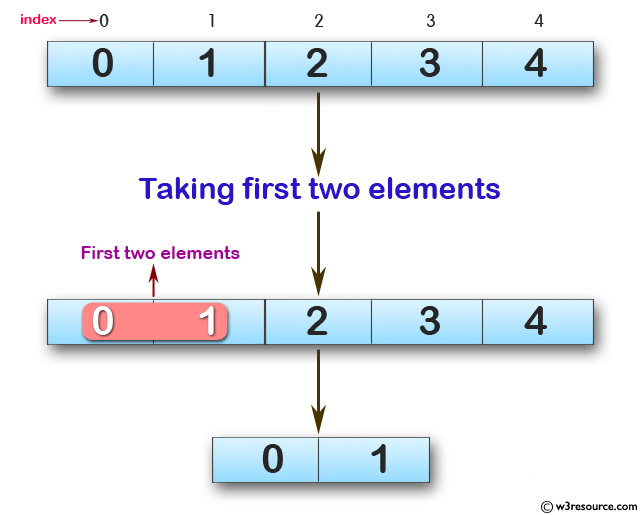﻿ Swift Array Programming Exercise: Create a new array, taking first two elements from a given array of integers - w3resource# Swift Array Programming Exercises: Create a new array, taking first two elements from a given array of integers

## Swift Array Programming: Exercise-22 with Solution

Write a Swift program to create a new array, taking first two elements from a given array of integers. If the length of the given array is less than 2 use the single element of the given array.

Pictorial Presentation:Sample Solution:

Swift Code:

``````func new_array(_ a: [Int]) -> [Int] {
guard a.count > 1 else
{
return a
}

return Array(a.prefix(2))
}
print(new_array([0, 1, 2, 3, 4]))
print(new_array([0, 1, 2]))
print(new_array())
```
```

Sample Output:

```[0, 1]
[0, 1]

```

Swift Programming Code Editor:

Improve this sample solution and post your code through Disqus

What is the difficulty level of this exercise?

﻿